# Raspberry Pi_Eng_25.4.4 Kirchhoff’s Laws

#### Published Book on Amazon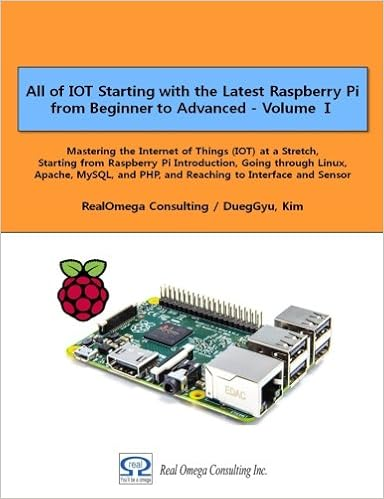All of IOT Starting with the Latest Raspberry Pi from Beginner to Advanced – Volume 1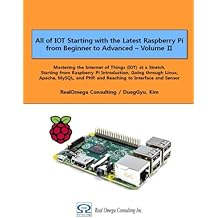All of IOT Starting with the Latest Raspberry Pi from Beginner to Advanced – Volume 2

#### 출판된 한글판 도서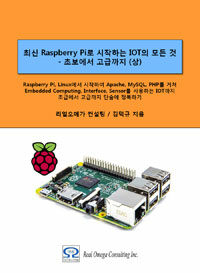최신 라즈베리파이(Raspberry Pi)로 시작하는 사물인터넷(IOT)의 모든 것 – 초보에서 고급까지 (상)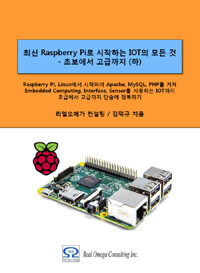최신 라즈베리파이(Raspberry Pi)로 시작하는 사물인터넷(IOT)의 모든 것 – 초보에서 고급까지 (하)

### 25.4.4Kirchhoff’s Laws

Kirchhoff's law has a first law of current and a second law of voltage.

#### 25.4.4.1 First Law - The Law of Current

The first rule is that on a certain path of current, the sum of incoming currents and the sum of outgoing currents is the same. That is, the sum of the current flowing into one point of the circuit and the current flowing out of the point is the same.

incoming current  = outgoing current

In the following, the current (I1) flowing into the point A and the current (I2, I3, I4) flowing out of the point A have the relation as follows.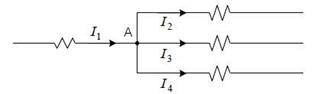I1  =  I2 + I3 +  I4

#### 25.4.4.2Second Law – The Law of Voltage

The second rule is that the sum of the supply voltage applied to the circuit and the sum of the voltage drop consumed in the circuit is equal

voltage supply = voltage drop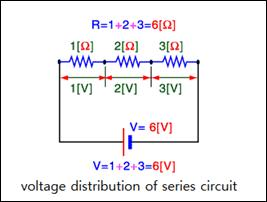In the above, the supply voltage applied in the circuit is divided into three resistances and consumed. That is, the load will be three resistances and a voltage drop will occur for each resistance. When all the voltage drop of each resistance is summed, it becomes the applied supply voltage.